# Noise in NC-AFM measurements with significant tip–sample interaction

1Fachbereich Physik, Universität Osnabrück, Barbarastraße 7, 49076 Osnabrück, Germany
2Department of Physics and Astronomy, The University of Utah, 115 South 1400 East, Salt Lake City, UT 84112-0830, USA
3now at: School of Physics & Astronomy, The University of Nottingham, University Park, Nottingham NG7 2RD, UK

1. Corresponding author email

Guest Editor: M. Z. Baykara
Beilstein J. Nanotechnol. 2016, 7, 1885–1904. https://doi.org/10.3762/bjnano.7.181
Received 20 Aug 2016, Accepted 28 Oct 2016, Published 01 Dec 2016

• Full Research Paper

## Abstract

The frequency shift noise in non-contact atomic force microscopy (NC-AFM) imaging and spectroscopy consists of thermal noise and detection system noise with an additional contribution from amplitude noise if there are significant tip–sample interactions. The total noise power spectral density DΔf(fm) is, however, not just the sum of these noise contributions. Instead its magnitude and spectral characteristics are determined by the strongly non-linear tip–sample interaction, by the coupling between the amplitude and tip–sample distance control loops of the NC-AFM system as well as by the characteristics of the phase locked loop (PLL) detector used for frequency demodulation. Here, we measure DΔf(fm) for various NC-AFM parameter settings representing realistic measurement conditions and compare experimental data to simulations based on a model of the NC-AFM system that includes the tip–sample interaction. The good agreement between predicted and measured noise spectra confirms that the model covers the relevant noise contributions and interactions. Results yield a general understanding of noise generation and propagation in the NC-AFM and provide a quantitative prediction of noise for given experimental parameters. We derive strategies for noise-optimised imaging and spectroscopy and outline a full optimisation procedure for the instrumentation and control loops.

Keywords: amplitude noise; cantilever stiffness; closed loop; detection system noise; frequency shift noise; non-contact atomic force microscopy (NC-AFM); Q-factor; spectral analysis; thermal noise; tip–sample interaction

## Introduction

Non-contact atomic force microscopy (NC-AFM) [1,2] is an unmatched surface science tool, especially when it comes to studying non-conducting surfaces [3,4], to map sub-molecular structures  or to measure forces  and force fields  with highest resolution. The primary imaging signal in NC-AFM is the frequency shift Δf of a probe resonator carrying a tip interacting with the sample surface , typically a cantilever, a tuning fork, or a needle sensor .

The resolution of force measurements is limited by the noise in the frequency shift signal [9,10], which strongly depends on the noise floor of the detection system, the frequency response of the frequency demodulator (mostly a phase-locked loop detector, PLL), cantilever properties and ultimately thermal noise . The footing of our work are these precursor studies, and the rigorous system analysis introduced by Polesel-Maris et al. , showing that the frequency shift noise at close tip–sample distance is increased due to a coupling of the phase-locked loop with the amplitude and the distance control loops.

While noise in the amplitude control loop itself is essentially independent of the frequency shift noise without tip–sample interaction, amplitude and topography feedback loop noise are coupled into the frequency shift noise in the presence of tip–sample forces . Ultimately, the noise in the frequency shift signal determines the base performance of all downstream processing such as the topography signal or the Kelvin probe force signal .

Here, we use the formalism derived by Polesel-Maris et al. , introduce realistic transfer functions for the control electronics, cantilever properties and tip–sample interaction, to quantitatively determine the frequency shift noise in the presence of significant tip–sample interaction, to derive predictions for noise spectra and to correlate them with experimental data obtained under realistic measurement conditions. We find excellent agreement between simulated and experimental results for noise in a cantilever-based NC-AFM with optical beam-deflection and measurements performed in an ultra-high vacuum environment, where the cantilever Q-factor is close to the intrinsic value Q0 [14,15]. Our analysis can, however, be applied to any NC-AFM detection scheme and sample environment, specifically also to measurements in liquids where signal-to-noise-ratio considerations play a paramount role [16-18]. From our findings, we derive a general strategy for adjusting instrumental settings and control loops for noise-optimised operation. A full glossary of all of these settings and further quantities relevant in this context are compiled in appendix A.

Our analysis is based on four fundamental steps: First, the cantilever oscillation amplitude is determined precisely by calibrating the voltage signal proportional to the cantilever displacement with a method described in detail elsewhere . This yields the detection sensitivity(see also Supplementary Information section 1 of ). Second,is used to convert the displacement noise voltage signal into the displacement noise quantities, namely the displacement noise power spectral densityof the detection system (frequently referred to as the noise floor) and the thermal noise power spectral density. Note that the latter cantilever thermal excitation noise contribution can be predicted from the oscillator properties and temperature . Third, the frequency response Hfilter of the PLL system is used for describing the propagation of noise from the cantilever oscillation to the frequency shift signal at the output of the frequency demodulator. This frequency response function strongly depends on the PLL filter settings  and will here be modelled for a typical experimental setup described in section “Noise propagation model” and appendix C. Fourth, we determine the explicit frequency response functions HA and Hz of the amplitude and topography control loops, respectively. This allows an adjustment of the amplitude control loop and the frequency response of the PLL prior to the measurement when tip–sample interaction is absent (i.e., with the tip retracted). The frequency response of the distance control loop, however, inherently depends on the tip–sample interaction which is, in turn, preset by the z-position along the force–distance curve . Therefore, this control loop needs adjustment under conditions of the envisaged measurement.

After describing experimental methods and procedures in section “Experimental”, we introduce the NC-AFM model used to simulate noise generation and propagation in section “Noise propagation model”. In section “Tip–sample interaction”, we then discuss the implications of the tip–sample interaction on the coupling of control loops. After a check of validity and consistency of the model by testing simulation results against measurements for the case of absent tip–sample interaction in section “Noise with negligible tip–sample interaction”, we systematically explore cases with significant tip–sample interaction in section “Noise with significant tip–sample interaction”. The investigation comprises measurements and simulations for scanning the surface at a constant tip–sample distance (constant-height mode) as well as with the frequency shift kept at a certain value by the z-control loop (topography mode). For the simulations, the filter settings of the control loops are varied over ranges of values typically present in experiments, and an artificial but realistic model potential is used for the tip–sample interaction. We validate the noise model including tip–sample interaction and describe a rational procedure for choosing system parameters for noise-optimised measurements in section “Conclusions and system optimisation”. All equations within this work are written using power spectral densities DX for the quantity X, while simulated and experimental results are described in terms of amplitude spectral densities.

## Experimental

All experiments are performed using a commercial NC-AFM system (UHV 750 variable temperature STM/AFM, RHK Technology, Inc., Troy, MI, USA) operated at room temperature and employing the beam-deflection method to measure the cantilever displacement. Tip positioning and approach is accomplished by the SPM 100 control system (RHK Technology, Inc.). For this and all other instruments we introduce scaling factors to convert voltage signals delivered by the instruments to physical units. The detection sensitivityfor the herein presented data is determined to 52.5 nm/V from an amplitude calibration (see  and Supporting Information of ). A PLLpro2 control system (RHK Technology, Inc., Troy, MI, USA) is used for frequency demodulation and amplitude stabilisation. This control system encodes the frequency shift Δf in volts using SΔf = −30 Hz/V. For the distance control loop, we employ a digital PI controller of the HF2LI device (Zurich Instruments AG, Zürich, Switzerland) as this instrument provides loop filters with well-defined characteristics. Noise measurements at the Δf and amplitude outputs of the PLL system as well as at the topography output of the distance controller are performed using a SR770 spectrum analyser (Stanford Research Systems, Inc., Sunnyvale, CA, USA). The topography signal is scaled using the sensitivity= 9.36 nm/V for the scanner z-piezo response. This value was determined from measuring step heights on CaF2 surfaces.

The cantilever is a commercial silicon cantilever (type PPP-NCH, Nanoworld AG, Neuchâtel, Switzerland) with an eigenfrequency of f0 = 305337.6 Hz at room temperature and a quality factor of Q0 = 43900 determined as described elsewhere . The noise floor is determined toand the modal stiffness of the cantilever  to k0 = 32.4 N/m from a measurement of the thermally excited cantilever oscillation  with the spectrum analyser of the HF2LI device. The cantilever oscillation is stabilised at an amplitude of A = 13.6 nm, which corresponds to an amplitude of Az = A cos(θ) = 12.6 nm perpendicular to the surface due to the inclination of θ = 22.5° between cantilever and sample surface given by the cantilever mount. These experimental parameters are used in all simulations presented within this work.

The tip–sample interaction modelled by the parameter βts (see section “Tip–sample interaction”) is derived from a measured Δf(zp) curve shown in Figure 4). Here, Δf is plotted against the piezo position zp. Depending on the operation mode (constant-height or topography), the parameter βts can be obtained by using either the frequency shift set-point Δfset for the topography feedback or by the average frequency shiftmeasured at the tip–sample distance zp with deactivated topography feedback loop.

For the numerical evaluation of signal vs time traces and noise spectra, the explicit frequency response functions and system parameters for our experimental setup are used; all frequency response functions are listed in the appendix and the implementation in MATLAB is available in Supporting Information File 1. This approach enables a numeric evaluation in absolute physical units and, therefore, allows the direct comparison between experiment and our model.

## Results and Discussion

### Noise propagation model

Figure 1 illustrates the signal and noise propagation in a typical NC-AFM setup. The cantilever is excited by a drive signal with frequency fexc and amplitude Aexc. Additionally, the cantilever experiences an excitation due to thermal noise expressed by the power spectral density= 2kBT/(k0Q0πf0). The cantilever responds to these excitations with an oscillation of amplitude A dictated by the cantilever response function Hc(f). This cantilever oscillation is measured as the cantilever displacement signal. Noise contributions in this signal are described in frequency space by the thermal noise displacement power spectral densityand by the detection system noise power spectral density, the latter caused by the electronic detection system . The sum of the detection system noise power spectral densityand the thermal displacement noise power spectral densityyields the total displacement noise power spectral density Dz(f).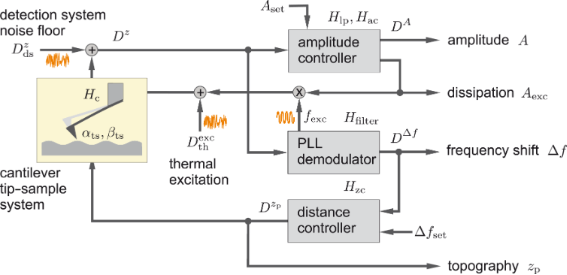Figure 1: Schematic representation of functional elements of an NC-AFM described by transfer functions Hy. Quantities DX denote noise power spectral densities of the signal X. Symbols “+” and “×” denote entry points of noise and entanglement of signals, respectively. Figure 1: Schematic representation of functional elements of an NC-AFM described by transfer functions Hy. Qu... Jump to Figure 1

The cantilever displacement signal is fed into both, the amplitude controller and the PLL demodulator. The amplitude controller measures the oscillation amplitude A typically using a root-mean-square algorithm or lock-in detection and adjusts the excitation amplitude Aexc to keep the oscillation amplitude A at the set-point Aset. The amplitude measurement includes a low-pass filter with the response function Hlp(f), while the amplitude controller is described by the frequency response Hac(f). Noise in the amplitude signal is characterised by the amplitude noise power spectral density DA(f).

The PLL demodulator determines the frequency shift Δf = frf0, which is the difference between the cantilever resonance frequency fr in the presence of tip–sample interaction and the cantilever eigenfrequency f0. Furthermore, the demodulator provides the cantilever excitation signal with frequency fexc that is nominally identical to the current resonance frequency fr of the cantilever. The frequency shift noise power spectral density DΔf(fm) depends on the filter and loop settings of the PLL demodulator expressed by its frequency response function Hfilter(fm), where fm represents the frequencies of the modulation side bands measured relative to the resonance frequency fr . Thus, the cantilever excitation signal contains noise from both, the PLL and the amplitude controller.

The frequency shift signal is fed into the distance controller, which adjusts the tip–sample distance to maintain a frequency shift equal to the set-point Δfset. The tip–sample distance is expressed as the position zp of the z-piezo (see below in Figure 3) and is in this context commonly referred to as the topography signal. The distance-dependent frequency shift Δf(zp) is governed by the details of the tip–sample interaction forces, and is herein for a few specific tip–sample distances characterised by the two parameters αts(zp) and βts(zp) as described in section “Tip–sample interaction”. These parameters determine how fluctuations in the oscillation amplitude and the tip–sample distance are coupled into the frequency shift signal.

The noise propagation model used for our simulations is based on the approach introduced in  and sketched in Figure 2. In contrast to the NC-AFM functional scheme shown in Figure 1, here we focus on the noise signal paths and transfer blocks relevant to the noise propagation. Furthermore, we investigate amplitude noise and frequency shift noise in two separate loops that are coupled via the tip–sample interaction. Effectively, this approach splits the signal into a purely amplitude-modulated component (controlled by the amplitude control loop) and a signal with pure frequency (or phase) modulation (controlled by the frequency control loop including the PLL) . This separation stems from the small intermixing strength of the two modulations, and will be justified here based on experimental evidence.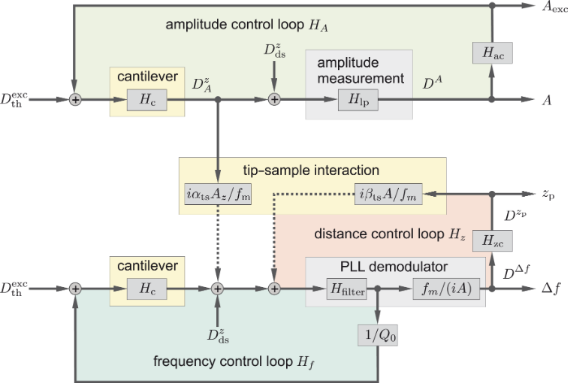Figure 2: Model for signal and noise propagation in an NC-AFM, highlighting the tip–sample interaction, PLL demodulator and control loops. Signal paths indicated by dotted lines are only relevant for the case of significant tip–sample interaction. Figure 2: Model for signal and noise propagation in an NC-AFM, highlighting the tip–sample interaction, PLL d... Jump to Figure 2

In the amplitude control loop (top part of Figure 2), the cantilever displacement signal contains amplitude fluctuations described by the noise power spectral densityand the detection system adds the noise floor, yielding the measured displacement noise Dz(f). The amplitude signal A follows from the displacement signal, which is then low-pass-filtered as described by the transfer function Hlp, and finally contains noise with the amplitude power spectral density noise DA(fm). This signal is fed into the amplitude controller described by the transfer function Hac, generating the excitation signal amplitude Aexc. The amplitude control loop is closed by feeding this signal to the cantilever. Note that with closing the loop, a fraction of the noise DA(fm) is fed back to the cantilever, added to the thermal noiseand filtered by the narrowband cantilever response function Hc(f).

In the frequency control loop (bottom part of Figure 2), the measured cantilever displacement signal is fed into the PLL demodulator yielding the frequency shift signal Δf as well as the excitation signal for the cantilever in the feedback path. The control loop within the PLL demodulator (not shown in Figure 2) is discussed in appendix C.2. In this frequency control loop feedback path, displacement noise propagating from the PLL to the cantilever excitation is weighted by the reciprocal of the quality factor Q0. This factor defines the ratio of the cantilever excitation signal to the oscillation amplitude at the cantilever resonance if neither amplitude noise nor amplitude disturbances are present, i.e., in absence of tip–sample interactions. The sum of excitation signal noise and thermal excitation noiseis band-pass-filtered by the cantilever response function Hc(f) and added to the detection system noise floor. The loop is closed by feeding this signal into the PLL. In the case of negligible tip–sample interaction, the noise in the frequency control loop is virtually independent from the settings of the other control loops shown in Figure 2, although we note that a coupling may become apparent if either of the loops is operated in an unstable ringing configuration. If significant tip–sample interaction is present, two more signals, one from the amplitude and a second from the distance control loop, are added before feeding the signal into the PLL demodulator as described below.

The distance control loop employs a controller with transfer function Hzc to regulate the frequency shift Δf from the PLL by adjusting the piezo position zp. The slope βts = ∂Δf/∂z of the frequency shift vs distance curve Δf(z) models the tip–sample interaction and is usually a non-linear function of zp. The frequency shift noise DΔf(fm) is converted to topography noiseby the action of the distance controller with transfer function Hzc. The topography noise is scaled by the tip–sample interaction transfer function iβtsA/fm and added to other noise contributions at the PLL demodulator input. The loop is closed across the PLL.

The coupling between the amplitude and the frequency control loops, which exists in the presence of significant tip–sample interaction, is modelled by a transfer function iαtsA/fm with αts = ∂Δf/∂A, acting on the amplitude noise. The resulting noise is one of the contributions at the PLL demodulator input and increases the frequency shift noise.

### Tip–sample interaction

The tip–sample interaction closes the distance control loop and it couples the amplitude control loop with the frequency control loop. Both connections can significantly increase the noise in the frequency shift Δf output signal compared to the case of negligible tip–sample interaction.

The transfer of fluctuations from the piezo position zp into the cantilever deflection signal by the distance control loop (see Figure 2) is described by the parameter βts. This parameter is defined as the gradient of the frequency shift signal Δf with respect to the tip–sample distance zts (see  and appendix D):

The parameter βts can be parameterised by either the z-position of the lower turning point zts (Equation 1) or using the piezo position zp (Equation 2), which is the centre position of the cantilever oscillation (see Figure 3). We explicitly include the amplitude dependency on the frequency shift Δf by including the oscillation amplitude component Az perpendicular to the sample surface. This dependency follows from the convolution of the interaction force with the weighting function due to the cantilever oscillation. For large oscillation amplitudes Az, the functional dependencehas been found . Hence, for a small variation δzp of the zp position, βts can straightforwardly be determined from the slope of the Δf(zp) curve at the working point as illustrated by the model curve in Figure 4. Obviously, βts strongly varies as a function of zp.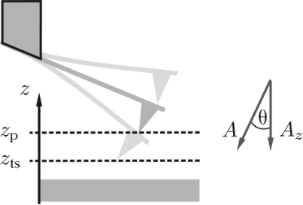Figure 3: Relations between the piezo position zp (tip position for resting cantilever), the lower turning point zts of the cantilever oscillation and the oscillation amplitude A as well as its projection Az on the sample surface normal. Figure 3: Relations between the piezo position zp (tip position for resting cantilever), the lower turning po... Jump to Figure 3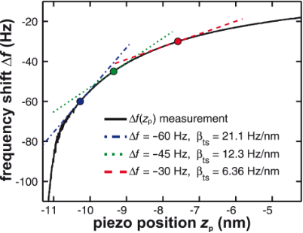Figure 4: Determination of the tip–sample interaction parameter βts from the slope of a measured Δf(zp) curve. Frequency shift data are plotted as a function of the z-piezo position zp. Figure 4: Determination of the tip–sample interaction parameter βts from the slope of a measured Δf(zp) curve... Jump to Figure 4

The parameter αts describes the transfer of cantilever deflection noisefrom the amplitude control loop into deflection noise in the frequency control loop via two mechanisms. First, a variation δA in the amplitude changes the weighting function in calculating the frequency shift from the cantilever oscillation  and, thus, the magnitude of the resulting Δf. Second, the variation leads to a shift of the lower turning point zts, bringing the sensor into a different tip–sample interaction regime. The coupling parameter αts is defined by 

For the experimental conditions within this work (see appendix D), small fluctuations δAz of the oscillation amplitude have the same effect as a small fluctuation δz in the center position, namely δz = −δAz. Therefore, we use the approximation

Further details on the relation between αts and βts assuming a model potential for the tip–sample interaction are provided in appendix D. Short-range forces acting between the probing tip and the sample surface are of primary relevance for our discussion as they typically exhibit strong gradients. Thus, the coupling strongly increases with increasing interaction when the tip is closely approached to the surface.

### Noise with negligible tip–sample interaction

We first analyse noise in the frequency shift Δf and amplitude A channel for the case of negligible tip–sample interaction (αts = βts = 0) to check for consistency with previous simulations  as well as experimental results . In respective experiments, we prepare this situation by retracting the tip several tens of nanometres from the sample surface.

The frequency shift noise power spectral densitystrongly depends on the PLL demodulator parameters  and is explicitly given by evaluating the frequency control loop in Figure 2 (see appendix B)

where the system parameters are those introduced in Figure 2. We use the explicit description of our experimental system (see appendix C for the individual frequency response functions) to numerically evaluate Equation 5. Note that the comparison of simulated noise spectra with experimental data is based on these system parameters and not on fitting.

The amplitude noise power spectral density DA is calculated from evaluating the amplitude control loop in Figure 2

We use the explicit system parameters for our experimental setup to evaluate Equation 6 numerically in absolute physical units.

In Figure 5, we compile measurements (solid lines) and simulations (dotted lines) for the frequency shift noise amplitude spectral density(panels (a) and (b)) and the amplitude noise amplitude spectral density dA (panels (c) and (d)). Panels (a) and (c) represent results for optimised amplitude loop gain settings while varying the PLL parameters. In contrast, panels (b) and (d) show results for optimised PLL parameters while varying the amplitude gain settings. In all data, the amplitude control loop filter Hlp has a 3rd-order Butterworth characteristics with a cutoff frequency of fc = 500 Hz.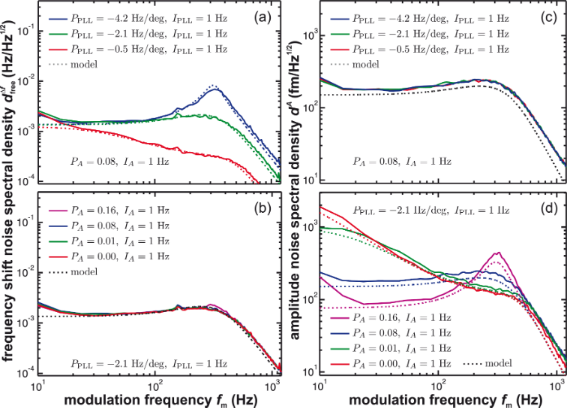Figure 5: Measured noise spectral density (solid lines) of (a, b) the frequency shift signal and (c, d) the amplitude signal for a variation of the proportional loop gain settings PPLL and PA of the PLL and amplitude control loop, respectively. The integral cutoff of the PLL loop (IPLL) and of the amplitude loop (IA) are each held constant. The tip is retracted from the surface for the measurements. Model calculations (dotted lines) based on Equation 5 and Equation 6 are performed assuming negligible tip–sample interaction. The loop filter Hlp has a 3rd-order Butterworth characteristics with a cutoff frequency of fc = 500 Hz, all other quantities are explicitly given in appendix C. Figure 5: Measured noise spectral density (solid lines) of (a, b) the frequency shift signal and (c, d) the a... Jump to Figure 5

Figure 5a demonstrates the low-pass filter characteristics of the PLL in the case of excessive filtering (low P-gain), optimum operating conditions (optimum P-gain) and gain peaking (high P-gain), respectively. The optimum frequency response is determined as described in appendix C, yielding optimum parameters of PPLL = −2.1 Hz/deg and IPLL = 1 Hz. Note that the PLL frequency response does not depend on the cantilever parameters. Thus, it can be optimised for the desired detection bandwidth by only considering the cantilever parameters. In contrast, the frequency shift noise at the PLL output generated by the frequency control loop depends on cantilever properties and several other system parameters including the PLL settings. The amplitude noise presented in Figure 5c is independent of the PLL loop settings and, similarly, the frequency shift noise shown in Figure 5b is independent of the amplitude loop settings, clearly demonstrating that the amplitude and frequency control loops are not coupled unless the PLL is operated in an unstable regime.

The spectral behaviour of the amplitude noise dA upon changing the amplitude control loop settings is slightly different from the behaviour observed in the frequency shift noiseupon changing the frequency control loop gain settings as demonstrated in Figure 5a and Figure 5d. When increasing the P-gain of the amplitude control loop, the noise in the low-frequency region decreases while in this case a peak around a frequency of about 300 Hz develops. If the amplitude control loop is disabled (PA = 0, red line), the noise spectral density becomes large in the low-frequency region as predicted by the simulation (dotted line). Thus, an activated amplitude control loop effectively compensates low-frequency noise in the amplitude signal. Optimum performance of this loop is obtained for the parameters PA = 0.08 and IA = 1 Hz using the criteria introduced in appendix C.

In conclusion, we find excellent quantitative agreement between simulated and experimental data for various settings of the amplitude and frequency control loop. The independence of the frequency shift noise (amplitude noise) upon changing the amplitude (frequency) control loop settings, respectively, clearly demonstrates the validity of separating the system in these two control loops as depicted in Figure 2 for the case of negligible tip–sample interaction.

### Noise with significant tip–sample interaction

Realistic NC-AFM imaging or force mapping experiments are performed at a small tip–sample distance, or even in the repulsive regime , where large gradients of the tip–sample force generate strong gradients in the frequency shift signal. We now extend Equation 5 and Equation 6 to include the additional noise contributions predicted by  and our system model in Figure 2.

The noise power spectral density of the cantilever oscillation amplitudeis not directly accessible experimentally, but can be introduced by analysing the amplitude control loop (see Figure 2)

The quantityitself is not affected by the tip–sample interaction. However, due to the coupling characterised by the parameter αts, the noise spectral densitypropagates into the frequency control loop, yielding a significant contribution to the frequency shift noise. From including this contribution in the control loop diagram of Figure 2, we find the frequency shift noise power spectral density DΔf as

Following the approach from the previous section, we use the explicit system parameters and system-specific transfer functions (given in appendix C) to numerically evaluate Equation 8 for comparison with the experimental data.

First, we investigate NC-AFM experiments performed in the constant-height mode, where the tip is in close proximity to the sample surface with the distance control loop disabled, modelled here by setting Hzc = 0. Measurements (solid lines) and corresponding simulations using Equation 8 (including tip–sample interaction, dotted lines) and Equation 5 (without tip–sample interaction, dashed lines) of the frequency shift noise spectral density DΔf are reproduced in Figure 6. Measurements and simulations are performed with enabled (PA = 0.08) and disabled (PA = 0) amplitude control loop as shown in Figure 6a and at two tip–sample distances characterised by the averaged frequency shiftas shown in Figure 6b. The increase in the spectral noise at low frequencies in Figure 6a indicates contributions from the cantilever amplitude noise coupling into the frequency shift signal via the tip–sample interaction. Despite some discrepancy at very low frequencies also observed for the case of negligible tip–sample interaction (see Figure 5), we find a good agreement between prediction and experimental results. Here, we can only speculate that the low-frequency deviation is caused by mechanical instabilities within the system, or by instabilities within the piezoelectric excitation system. For example, low-frequency noise has been observed when using photothermal excitation .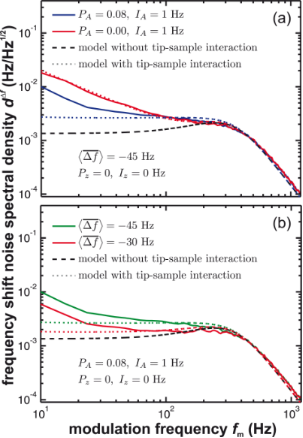Figure 6: Frequency shift noise spectral density dΔf for the case of significant tip–sample interaction measured in the constant height mode (Pz = 0, Iz = 0 Hz) in dependence on (a) the amplitude control loop settings and (b) the tip–sample distance parametrised via the averaged frequency shift . Measured curves (solid lines) are compared to model predictions including tip–sample interaction (dotted lines, Equation 8) and to the model without tip–sample interaction (dashed lines, Equation 5). The loop filter Hlp has a 3rd-order Butterworth characteristics with a cutoff frequency of fc = 500 Hz. Figure 6: Frequency shift noise spectral density dΔf for the case of significant tip–sample interaction measu... Jump to Figure 6

Disabling the amplitude control loop results in a strong increase of low frequency noise compared to operation with engaged amplitude control using optimum parameters (see previous section and appendix C). The amplitude control loop effectively reduces the frequency shift noise by its negative feedback. Furthermore, we observe an increase of the frequency shift noise DΔf for stronger tip–sample interaction (see Figure 6b) due to a strong coupling described by an increase of αts at smaller tip–sample distances.

Second, we investigate the frequency shift and topography noise in the commonly used constant frequency-shift mode where the tip–sample distance is adjusted by the distance control loop to keep the frequency shift at the set-point Δfset. The topography noise spectral densityis obtained by applying the frequency response Hzc of the distance controller to the frequency shift noise DΔf (see Figure 2)

Figure 7 shows the measured frequency shift (panels (a, b)) and topography (panels (c, d)) noise in the presence of the activated distance control loop (solid lines). These experimental data are compared to simulation results based on Equation 8 including tip–sample interaction (dotted lines) and Equation 5 without tip–sample interaction (dashed lines).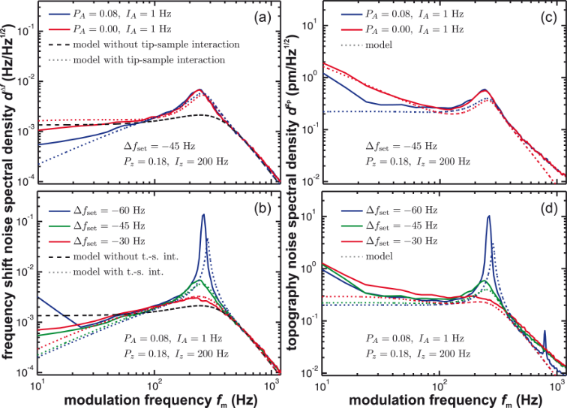Figure 7: (a, b) Frequency shift noise spectral density dΔf and (c, d) topography noise spectral density with tip–sample interaction using the constant frequency-shift mode in dependence on the amplitude control loop settings and the tip–sample distance defined by the frequency shift set-point Δfset. Measured curves (solid lines) are compared to model predictions including tip–sample interaction (dotted lines, Equation 8 and Equation 9) and without tip–sample interaction (dashed lines, Equation 5). The loop filter Hlp has a 3rd-order Butterworth characteristics with a cutoff frequency of fc = 500 Hz. Figure 7: (a, b) Frequency shift noise spectral density dΔf and (c, d) topography noise spectral density wit... Jump to Figure 7

Generally, we observe an increase of noise power in the frequency range from 200 to 300 Hz, where the apparent peaking firsthand appears to be independent from the amplitude control loop settings. Engaging the amplitude control loop (Figure 7a and Figure 7c) results in a reduction of noise in the low-frequency regime as observed in the constant-height measurement mode. However, the active loop has a marginal influence on the peaking in the 200–300 Hz region. In contrast, the appearance of this peak strongly depends on the frequency shift set-point: The peak height increases with increased frequency shift set-point Δfset (Figure 7b and Figure 7d). Interestingly, with activated distance control, the noise level in the low-frequency range may even fall below the values observed without tip–sample interaction. This demonstrates that the distance control loop is effectively able to compensate some of the low-frequency thermal noise by a distance adjustment and directly suggests an optimum frequency response as outlined in appendix C.

Finally, we investigate in Figure 8 the influence of the distance control loop parameters Pz and Iz on the noise characteristics. Frequency shift noise dΔf (panels a and c) and topography noise(panels c and d) experimental data (solid curves) are compared to simulations based on Equation 8 including tip–sample interaction (dotted lines) and Equation 5 without tip–sample interaction (dashed lines). In all cases, the amplitude control loop is set using optimum parameters. Data in Figure 8 are presented for different Pz (Iz) while keeping Iz (Pz) constant, respectively. Choosing the gain factors too large results in gain peaking in the noise spectral density of the frequency shift signal as well as the topography signal. Different Pz (Figure 8a and Figure 8c) shift the gain peak along the frequency axis and we find in this example a minimum of the peak amplitude for Pz = 0.18. This behaviour is further illustrated by discussing the frequency and step response of the distance control loop in appendix C. Decreasing Iz (Figure 8b and Figure 8d) reduces the gain peaking but elevates the noise level in the low-frequency range of the frequency shift noise. In the topography noise, a decrease of Iz significantly reduces the total noise. This effect is not surprising as it coincides with a reduction of the gain in the frequency range around 100 Hz and a significant slow-down of the step-response as shown in Figure 12 of appendix C. A small response time of the topography feedback loop causes a reduced noise in.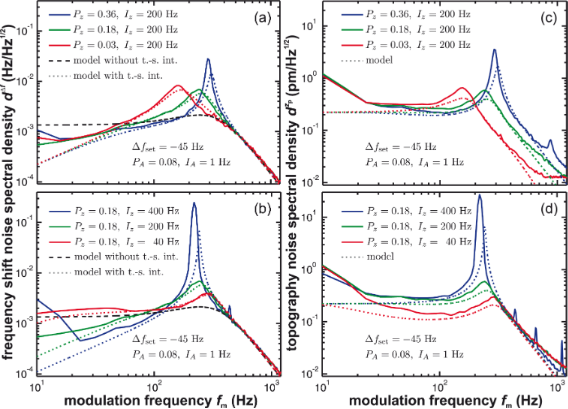Figure 8: (a, b) Frequency shift noise spectral density dΔf and (c, d) topography noise spectral density with tip–sample interaction in the constant frequency-shift mode in dependence on the distance control loop settings. Measured curves (solid lines) are compared to model predictions including tip–sample interaction (dotted lines, Equation 8 and Equation 9) and without tip–sample interaction (dashed lines, Equation 5). The loop filter Hlp has a 3rd-order Butterworth characteristics with a cutoff frequency of fc = 500 Hz. Figure 8: (a, b) Frequency shift noise spectral density dΔf and (c, d) topography noise spectral density wit... Jump to Figure 8

## Conclusions and System Optimisation

We realise that the control and data acquisition system of a NC-AFM is a complex network of sensing, amplification and processing stages as well as several control loops interacting with each other. Our network analysis demonstrates the quantitative description of all frequency response functions of the NC-AFM system, including the prediction of noise confirmed by an excellent agreement between measurement and network modelling. This analysis especially provides experimental evidence for strong noise amplification by coupling of control loops due to the tip–sample interaction.

In regular NC-AFM operation with state-of-the-art hardware, signal generation and noise amplification is governed by the tip–sample interaction, which introduces the most non-linear transfer function into the system. Therefore, the optimisation of NC-AFM measurements by proper settings for system parameters is not straightforward and has to be carefully adapted to the specific measurement task. Often, corrections are necessary during measurements upon a change in tip–sample interaction, for instance due to a change in tip–sample distance or a tip change. In such situations, best results are commonly obtained by following the instinct of the experienced experimentalist.

However, the basic adjustment of the system to yield the optimum in stability, accuracy and signal-to-noise ratio can be done by a rational, systematic approach following the findings described in this paper, provided the measurement system is well characterised and offers sufficient choice and flexibility in system parameter settings.

The starting point is always the experimental task defining the desired spatial resolution λ that is, for instance, a fraction of the atomic periodicity in atomic resolution imaging, and the available time for the measurement expressed by the scan speed vscan. Assuming perfectly band-limited output signals, the sampling theorem requires the product of scan speed and inverse spatial resolution to be smaller than half of the detection bandwidth ΔfBW, or

This often requires a compromise as using the optimum bandwidth defined by operation at the thermal noise limit  may impose a scan speed that is not practical, specifically if thermal drift is not compensated . Considering the interdependence of the control loops and the tip–sample interaction, we suggest four optimisation steps to be performed in following order: (1) the PLL demodulator Hfilter, (2) the frequency control loop Hf, (3) the amplitude control loop HA and (4) the distance control loop Hz.

In step (1), the PLL demodulator Hfilter is optimised purely from simulating the frequency response to have a certain bandwidth ΔfBW. For an integral cutoff IPLL of the PLL loop given from the ratio f0/Q0  and for a low-pass filter Hlp selected according to the requested bandwidth ΔfBW, the feedback gain parameter PPLL is increased until the peak threshold of 0.1 dB is reached (see appendix C.2 for an example and further details). As it is most desirable to work with high-Q cantilevers , the frequency control loop Hf is in step (2) inherently optimised from step (1) (see appendix C.2 for details). In case of small Q values (i.e., in liquid environment ), the optimisation in step (2) can be performed from simulating the frequency response with the knowledge of the system parameters f0 and Q. Optimising the amplitude control loop response HA in step (3) requires the cantilever parameters f0 and Q0 that can easily be determined . Here, the integral cutoff IA of the amplitude loop is again set to f0/Q0 and the feedback gain parameter PA is then increased until the threshold of 0.1 dB for gain peaking is reached as outlined in appendix C.1. This optimisation can also be performed purely from simulating the frequency response function. In step (4), the frequency response of the distance control loop is optimised. This requires the acquisition of a Δf(zp) curve, from which the slope βts is calculated at the working point. The feedback loop gains Pz and Iz are optimised until an acceptable overshoot and a fast step response is achieved as outlined in appendix C.3. Due to the usually imminent risk of tip changes, it is advisable to plan with a safety buffer regarding these two parameters.

Specifically the last step is most crucial and requires utmost care, not only in experiment preparation but also during the experimental run. Following the outlined procedure will yield the best possible result. If this is not satisfactory, the reason is often that the base value ofis too high or that the detection system noise contains disturbing signals, such as radio frequency interference or spurious cantilever excitation. Therefore, it is always good practice to additionally check the measurement signal with a spectrum analyser from the pre-amplifier all way down to the PLL output. The quality of measurements may dramatically be increased by removing even a minute spurious signal generated at a critical frequency to avoid its amplification by the system network. In this case, our optimisation procedure can bring the NC-AFM setup to noise-optimised performance.

## Appendix

### A Glossary

Table 1 is a glossary of all symbols used within this work to parametrise noise in an NC-AFM system.

### B Frequency response of control loops

We briefly outline how we calculate a closed loop response Hxy for a loop containing frequency response functions Hi between the input signal X and the output signal Y. For a more detailed discussion we refer to . All frequency response functions are treated as a function of the complex frequency iω. In the main text, we mostly evaluate the real component with respect to f = ω/(2π) (or fm) as this “amplitude response” or “gain” can directly be compared to experimental data. Furthermore, we usually do not consider the signal phase in this work, as we are interested in noise that is a result of stochastic processes. However, the frequency response functions Hi(iω) are treated as transfer functions Hi(s) using the complex frequency variable s = σ + iω to calculate step responses from an inverse Laplace transformation.

Figure 9a is a block diagram of the frequency and distance control loop of Figure 2. The model contains two closed loops that are interlaced. Using the corresponding signal-flow graph in Figure 9b and Mason’s theorem , we are able to describe the interlaced feedback loops by one transfer function. While the block diagram in Figure 9a focuses on the involved transfer functions, the signal-flow graph in Figure 9b represents the topological structure of the system. After using basic signal-flow graph algebra  and following the analysis introduced by Shinners , this signal-flow graph directly permits to derive a solution for the transfer function.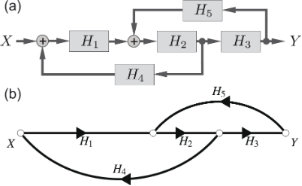Figure 9: (a) Block diagram of interlaced control loops as introduced in Figure 2 and (b) signal-flow graph to demonstrate the derivation of the frequency response of coupled closed loops. Figure 9: (a) Block diagram of interlaced control loops as introduced in Figure 2 and (b) signal-flow graph to demons... Jump to Figure 9

According to Mason’s theorem, the general expression for the signal-flow graph frequency response Hxy is

where Δ is the determinant of the graph defined as

withWe exemplarily calculate the full frequency response for the system sketched in Figure 9 where only one forward path (H1H2H3) is in the corresponding signal-flow representation. Therefore, the calculation reduces to determineand Δ1. Furthermore, two non-touching closed loops, namely H1H2H4 and H2H3H5, are present. Consequently, Δ is reduced toand reads

Evaluating

and

allows us to determine the full frequency response from X to Y from Figure 9 as

If a noise power spectral density Dx is used as the input signal X and treated by the system response Hxy we find

for the output noise power spectral density Dy .

### C Frequency response functions

In this section, we present the explicit form of the frequency response functions and the specific frequency response functions valid for the experimental setup used for this work. The derivation follows  and .

#### C.1 Amplitude control loop

The frequency response Hac of the amplitude controller is 

using the amplitude and excitation calibration factorsand, respectively, whereis determined by an amplitude calibration as described in  whileis determined from measuring the oscillation amplitude in resonance for a given excitation voltage Vexc. Assuming Q0 = A/Aexc =allows a straightforward calculation offrom known parameters of a well-characterised system. Note that by rewriting this formula to, we can fully describe Hac without performing an amplitude calibration measurement. The characteristics of the loop are defined by two parameters, the gain PA and the integral cutoff IA. Assuming that the cantilever is a system of first order, the integral cutoff IA can be chosen to f0/Q0 to avoid loop instabilities . Therefore, the formula is written directly in terms of the integral cutoff and not using the integral loop gain I = IAPAπ.

The frequency response of the cantilever follows from the response of a damped harmonic oscillator

with the quality factor Q0 and the eigenfrequency ω0 = 2πf0. This function can be re-written with the modulation frequency ωm as the argument by substituting ω = ω0 + ωm and can forbe approximated  to

Note that Equation 20 is phase shifted by π/2 relative to Equation 19 . Following procedures outlined in appendix B and Figure 2, the frequency response of the closed amplitude control loop is given as

with the frequency response of the amplitude controller Hac (see Equation 18), the frequency response of the cantilever Hc (see Equation 20) and the frequency response of the loop filter Hlp, which is in our case a Butterworth filter of 3rd order  with cutoff frequency fc

To quantitatively evaluate HA, we first note that Hc is fully defined by the two cantilever parameters f0 and Q0. These can easily be determined in absence of tip–sample interaction . By adding the response functions of the loop filter and amplitude controller according to Equation 21, we calculate the frequency response of the amplitude control loop illustrated in Figure 10a for different proportional gain values PA. Figure 10b shows the corresponding step response in time space, which is calculated by applying the inverse Laplace transform  to the product of the transfer function HA(s) with s = σ + iω and the Laplace transform of the unit step function, 1/s. The resultis numerically evaluated . The PLLpro2 system provides a feedback test by periodically changing one parameter, here the amplitude set-point, by a given magnitude, while recording the respective response with time. The measurements are normalised to a step height of one to be comparable to the calculated step responses. As shown in panel (c), the calculations are in excellent agreement with the measured step response. The response functions follow the expected behaviour: For small PA, the frequency response is a decreasing function of frequency and the step response a slowly rising function in time (red curves). For large PA, gain peaking appears in the frequency response and the step response exhibits ringing (blue curves). The optimum setting is represented by the frequency response being flat over the low pass filter bandwidth followed by a steep decrease. This corresponds to a nearly rectangular step response with a certain rise time and a small overshoot (green curves).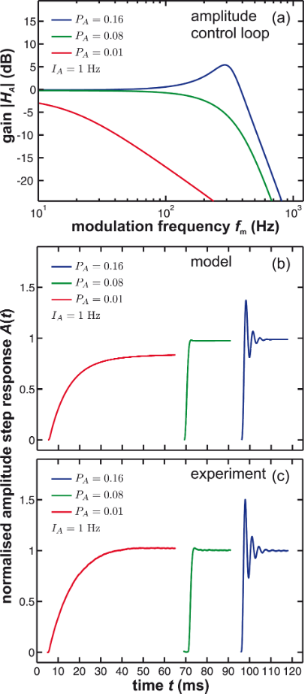Figure 10: (a) Calculated gain and (b) calculated step response of the amplitude control loop compared to (c) measured step response for different loop gains PA. IA is kept fixed at 1 Hz. The loop filter Hlp has a 3rd-order Butterworth characteristics with a cutoff frequency of fc = 500 Hz. Figure 10: (a) Calculated gain and (b) calculated step response of the amplitude control loop compared to (c) ... Jump to Figure 10

To optimise the amplitude control loop parameters, we first set the integral cutoff IA of the amplitude controller to f0/Q0 . To analyse the frequency response, we then start with a small PA and increase this value stepwise until a certain threshold for the gain peaking is reached, e.g., 0.1 dB. For the given set of parameters, this response reflects the optimum settings.

#### C.2 Frequency control loop

The frequency response of the PLL is given by (see Supplemental Information of )

with Hlp(iωm) being the frequency response of the low-pass loop filter. Figure 11a illustrates the calculated gain of the PLL using Equation 23 for different loop gain settings PPLL and a 3rd-order Butterworth filter (see Equation 22) with a cutoff frequency of 500 Hz. We experimentally observe that the integral cutoff IPLL has a minor influence on the frequency response besides the presence of gain peaking for very small values. In Figure 11b, the step response of Hfilter is calculated and compared to the measured step response of the PLLpro2 system shown in Figure 11c. Here, the inverse Laplace transform is used to calculate the frequency shiftand the PLLpro2 feedback test is experimentally performed by periodically changing the phase setpoint within the PLLpro2 frequency control loop while logging the frequency shift signal.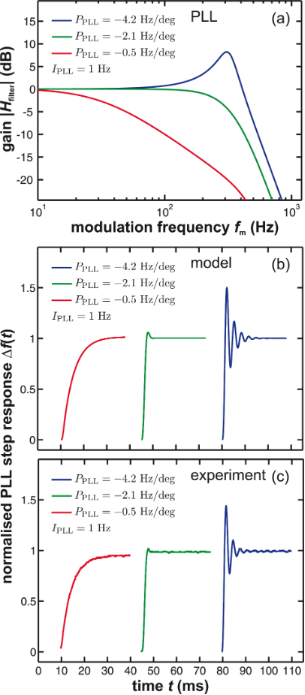Figure 11: (a) Calculated gain and (b) calculated step response of the PLL compared to (c) the measured step response for different loop gains PPLL. IPLL is kept fixed at 1 Hz. The loop filter Hlp has a 3rd-order Butterworth characteristics with a cutoff frequency of fc = 500 Hz. Figure 11: (a) Calculated gain and (b) calculated step response of the PLL compared to (c) the measured step r... Jump to Figure 11

For the closed frequency control loop, we find from Figure 2 and using procedures outlined in appendix B

By using Equation 20, we find Hc/Q0→0 for large Q0. As high Q0 values are always desirable in experiments performed under UHV conditions, this analysis suggests an optimisation procedure for the frequency control loop solely based on the frequency response Hfilter of the PLL. This optimisation is possible from calculating the gain before the cantilever is inserted into the system if the system parameters f0 and Q0 are known. The procedure can be performed similar to the optimisation of the amplitude loop, by first setting the integral cutoff IPLL to f0/Q0 and then increasing PPLL until the threshold of 0.1 dB for gain peaking is reached. Calculated and experimentally measured frequency and step response functions are acquired as before in case of the amplitude control loop and are presented in Figure 11.

#### C.3 Distance control loop

The the z-distance controller is a general proportional–integral regulator with frequency response

The voltage output of the PLL and the signal input of the piezos are both scaled in units of volts. To account for the correct unit of the frequency response function, we include a calibration factor SΔf (in units of Hz/V) for the PLL output and a calibration factor(in units of nm/V) as z-piezo sensitivity.

The frequency response of the closed distance control loop is determined from Figure 2 and using procedures outlined in appendix B

with Hzc being the frequency response of the distance controller (see Equation 25) and Hfilter being the frequency response of the PLL (see Equation 23). Figure 12a,b illustrate the calculated response of the distance control loop using Equation 26 for different settings of the proportional gain Pz and the integral gain Iz. The corresponding calculated step response of the distance control loop is shown in Figure 12c,d.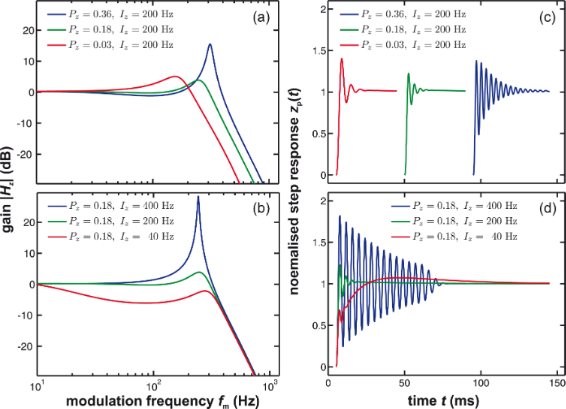Figure 12: (a, b) Frequency response and (c, d) step response of the distance control loop for a given tip–sample interaction βts = 12.3 Hz/nm and different settings of Pz and Iz. The calculations are performed using the ratio = −0.312 nm/Hz and for PLL settings PPLL = −2.1 Hz/deg, IPLL = 1 Hz with the loop filter Hlp having a 3rd-order Butterworth characteristics with a cutoff frequency of fc = 500 Hz. Figure 12: (a, b) Frequency response and (c, d) step response of the distance control loop for a given tip–sam... Jump to Figure 12

Figure 12 illustrates that a proper adjustment of the distance controller parameters Pz and Iz is mandatory for stable and fast operation. Compared to the previous loop discussions, a significant complication added is the parameter βts, which strongly depends on zp. Therefore, a configuration identified as the optimum for a certain tip–sample distance is most likely obsolete for stronger or weaker tip–sample interaction and would yield creep or overshoot in the step response.

For the optimisation of the distance control loop, a Δf(zp) curve should be obtained first and the slope of the Δf(zp) curve at the desired working point (βts = 12.3 Hz/nm, see Figure 4) should be used to simulate the frequency response of the distance control loop. As shown in Figure 12a and Figure 12c, an optimum for the lowest gain peaking could be found for Pz = 0.18 (green curve). When changing the integral gain Iz (see Figure 12b and Figure 12d), we start in this case from a strongly damped response (red curve), pass the optimum (Iz = 200 Hz, green curve) and arrive at a ringing behaviour (blue curve). The optimum is characterised by acceptable overshoot. A fast step response is obtained by reducing gain peaking while maintaining a flat response at low frequencies. However, operating on a slightly different position on the Δf(z) curve may strongly change the frequency response of the distance control loop. Therefore, it is advisable to plan with a safety buffer regarding the choice of Pz and Iz values to be prepared for unexpected changes of the tip–sample interaction.

### D Relation between αts and βts

The theory derived by Polesel-Maris et al.  describes the impact of the tip–sample interaction on the measurement signal noise by the two parameters αts and βts defined as

and

Here, zts denotes the z-position of the lower turning point of the cantilever oscillation (see Figure 3). This position is related to the piezo position zp and the oscillation amplitude Az by

Following an amplitude change of δA, the lower turning point shifts to zp − δA while the centre of the oscillation zp remains fixed. As a consequence of Equation 29, we find an identity for the derivations with respect to zts and zp:

Using this identity and Equation 29, we rewrite Equation 27 and Equation 28:

Thus, the parameter βts can be determined directly from the slope of a known Δf(zp) curve as shown in Figure 4. Furthermore, Equation 31 can be rewritten into two terms

This representation explicitly presents the two effects of an amplitude change on the frequency shift: First, the frequency shift changes due to a different lower turning point (αts,1) and, second, the change in Δf due to a change of the weighting function  in the Δf calculation (αts,2).

The first term is a measure of the slope of the Δf(zp) curve with respect to the piezo position zp. It is identical to −βts. The second term is a result from the convolution of the tip–sample force interaction with a weighting function . For large oscillation amplitudes, a dependencehas been found, allowing the definition of an amplitude-independent, normalised frequency shift . This second term becomes negligible for large oscillation amplitudes Az.

We illustrate the latter point by using an analytic expression for a Morse interaction force

for which the resulting frequency shift ΔfM can be calculated as 

where In(z) is the modified Bessel function of the first kind. Using this expression, parameters αts and βts are directly calculated as

The statement αts,1 = −βts is directly evident from Equation 37 and Equation 39. To quantify the relation between αts,1 and αts,2, we plot the ratio

as a function of the amplitude A and the lower turning point position zts in Figure 13 and using parameters for a Si–Si interaction derived from theory , namely Eb = 2.273 eV, κ = 12.76 nm−1 and σ = 0.2357 nm. Even at z-positions close to the force minimum (zmin ≈ 0.3 nm) and for amplitudes A larger than 5 nm, the parameter αts,2 is less than 5% of αts,1. Thus, under these conditions, the approximation

is fully justified.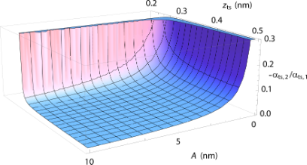Figure 13: Ratio δα = −αts,2/αts,1 as a function of the z-position and the amplitude. A Morse interaction using parameters from  and f0 = 300 kHz, k = 35 N/m are used to model the tip–sample interaction. Figure 13: Ratio δα = −αts,2/αts,1 as a function of the z-position and the amplitude. A Morse interaction usin... Jump to Figure 13

## Acknowledgements

We thank Steffen Porthun (RHK) and Sadik Hafizovic (Zurich Instruments) for most helpful discussions regarding the system-specific frequency response functions. M.T. gratefully acknowledges support from the Hans-Mühlenhoff-Stiftung. P.R. gratefully acknowledges support from the Alexander von Humboldt foundation. The project has been generously supported by Nanoworld Services GmbH.

#### Article is part of the thematic issue

##### Noncontact atomic force microscopy III

Mehmet Z. Baykara and Udo D. Schwarz

#### Interesting articles

##### Direct measurement of surface photovoltage by AC bias Kelvin probe force microscopy

Masato Miyazaki, Yasuhiro Sugawara and Yan Jun Li

##### Quantitative dynamic force microscopy with inclined tip oscillation

Philipp Rahe, Daniel Heile, Reinhard Olbrich and Michael Reichling

##### Measurement of electrostatic tip–sample interactions by time-domain Kelvin probe force microscopy

Christian Ritz, Tino Wagner and Andreas Stemmer

#### News

##### ROR allows to connect researchers and their outputs to affiliations##### Register for the Beilstein Nanotechnology Symposium 2022!##### We are looking for an active researcher who is interested in a unique opportunity with a non-profit diamond open access journal.This is an Open Access article under the terms of the Creative Commons Attribution License (http://creativecommons.org/licenses/by/4.0), which permits unrestricted use, distribution, and reproduction in any medium, provided the original work is properly cited.
The license is subject to the Beilstein Journal of Nanotechnology terms and conditions: (http://www.beilstein-journals.org/bjnano)